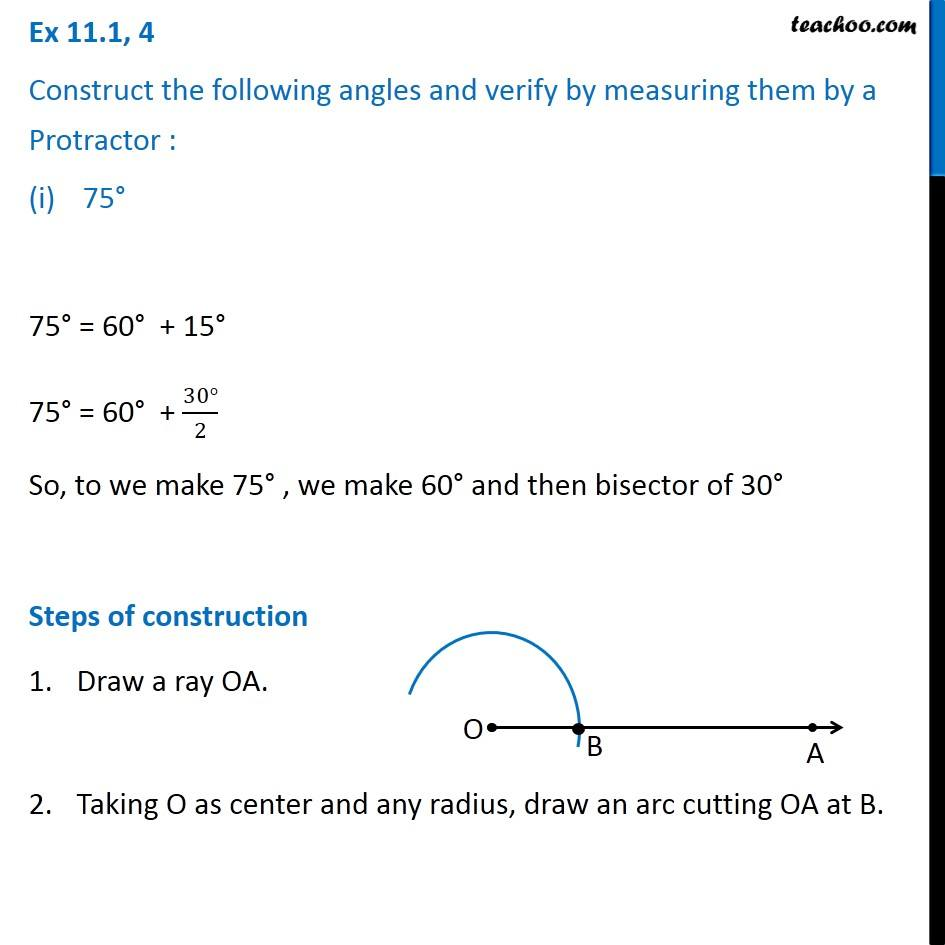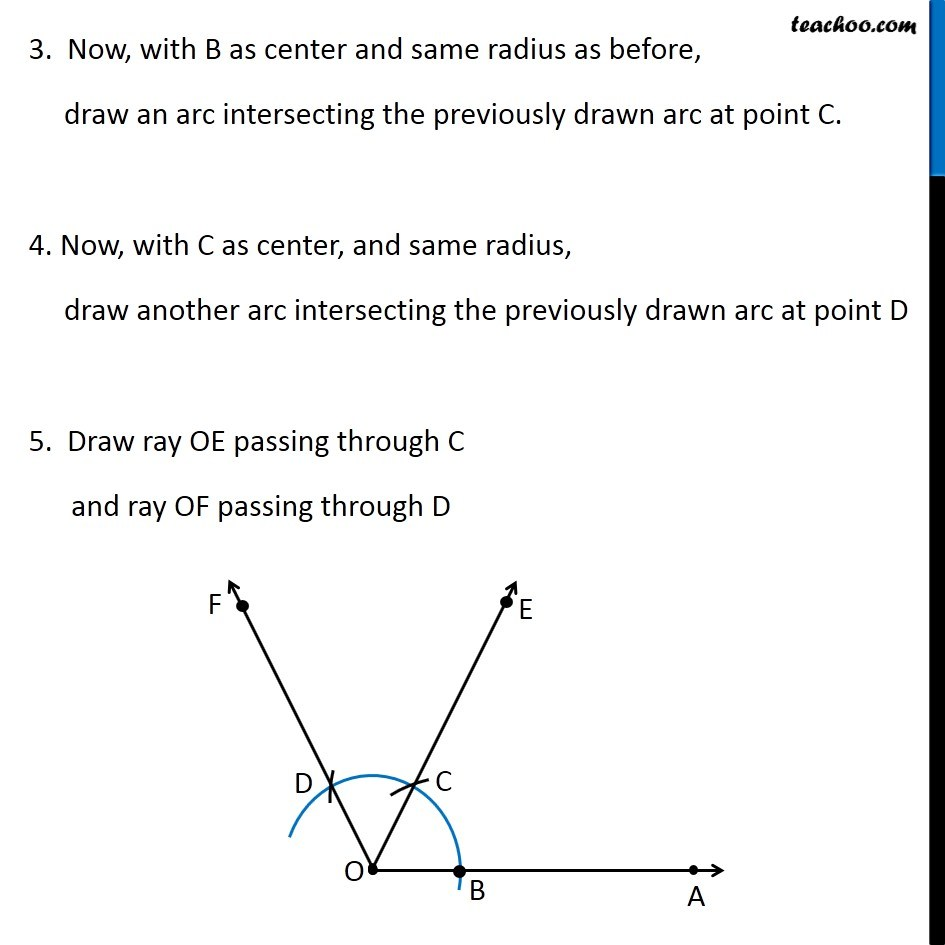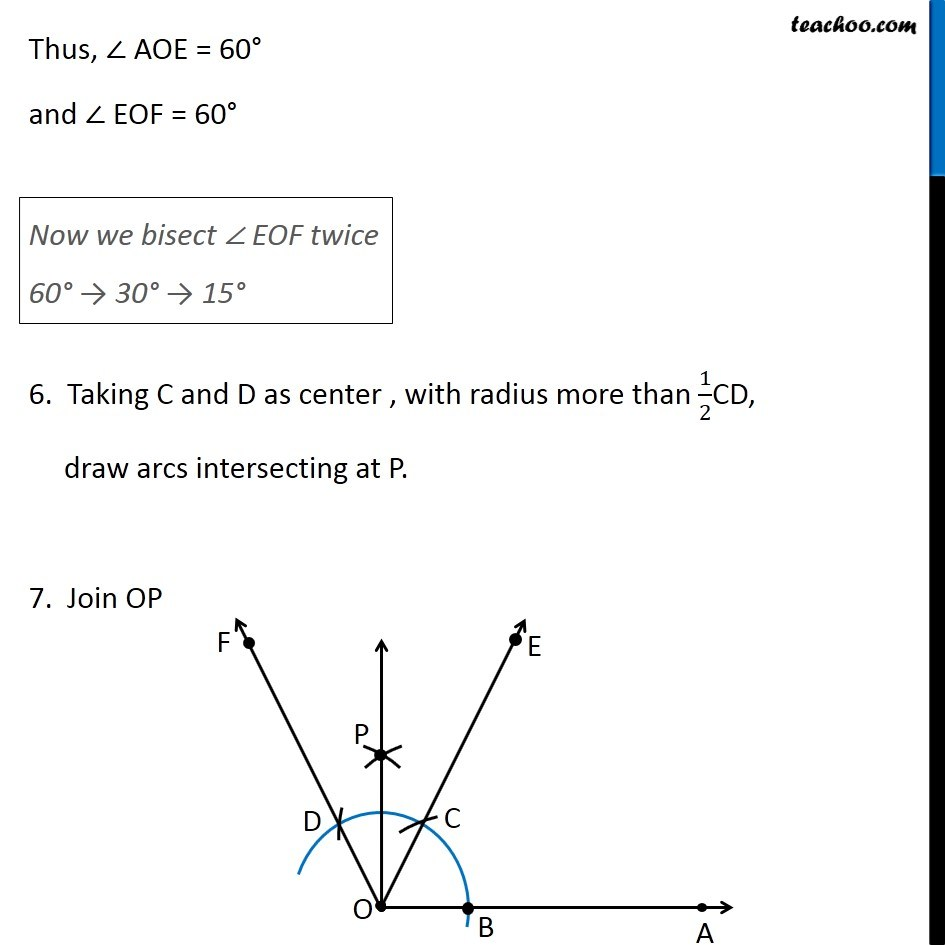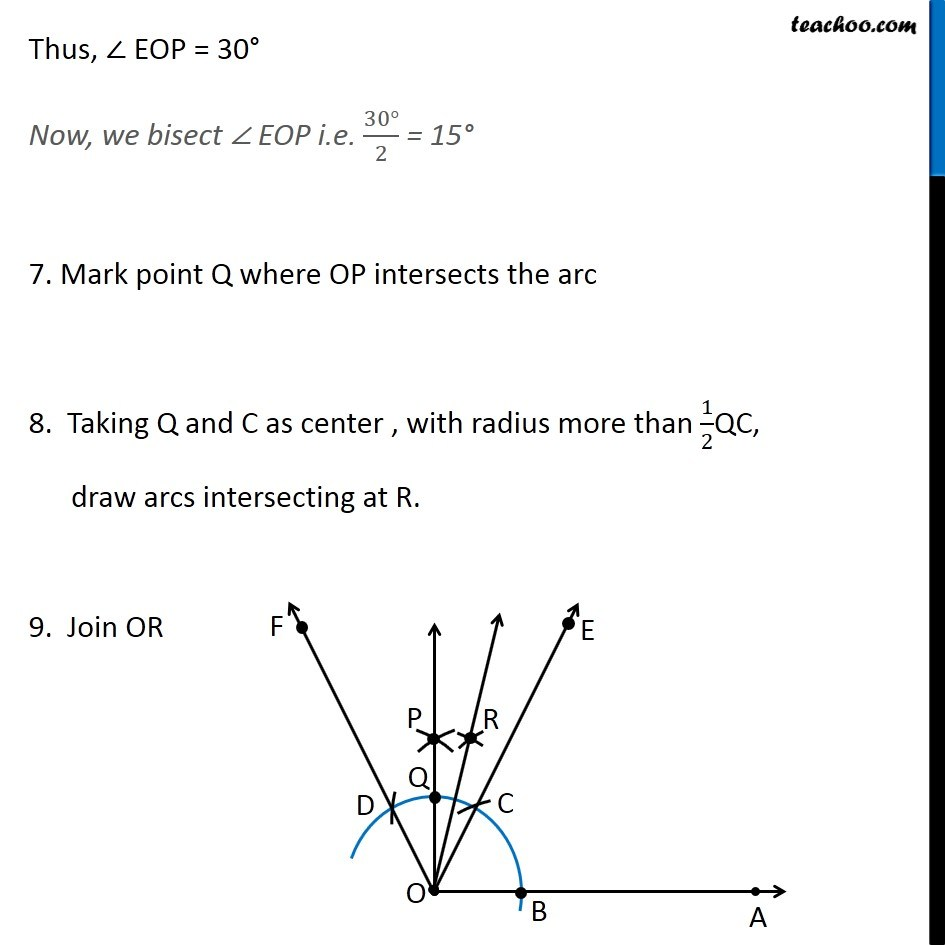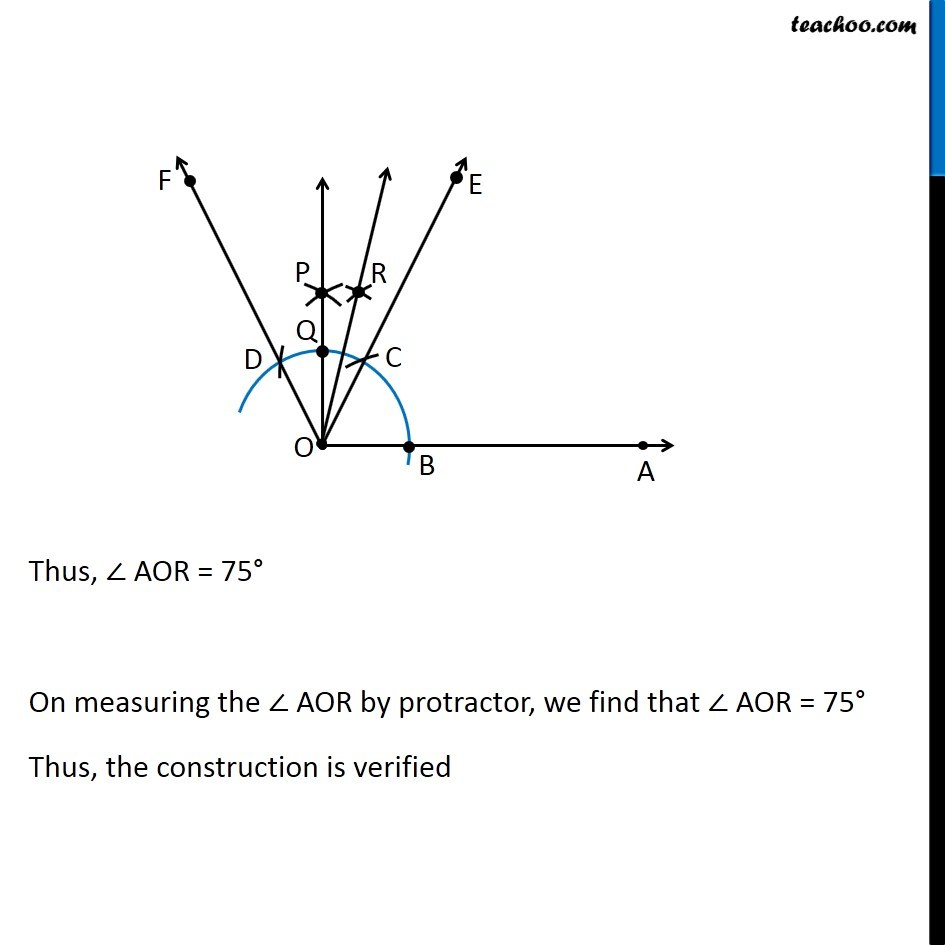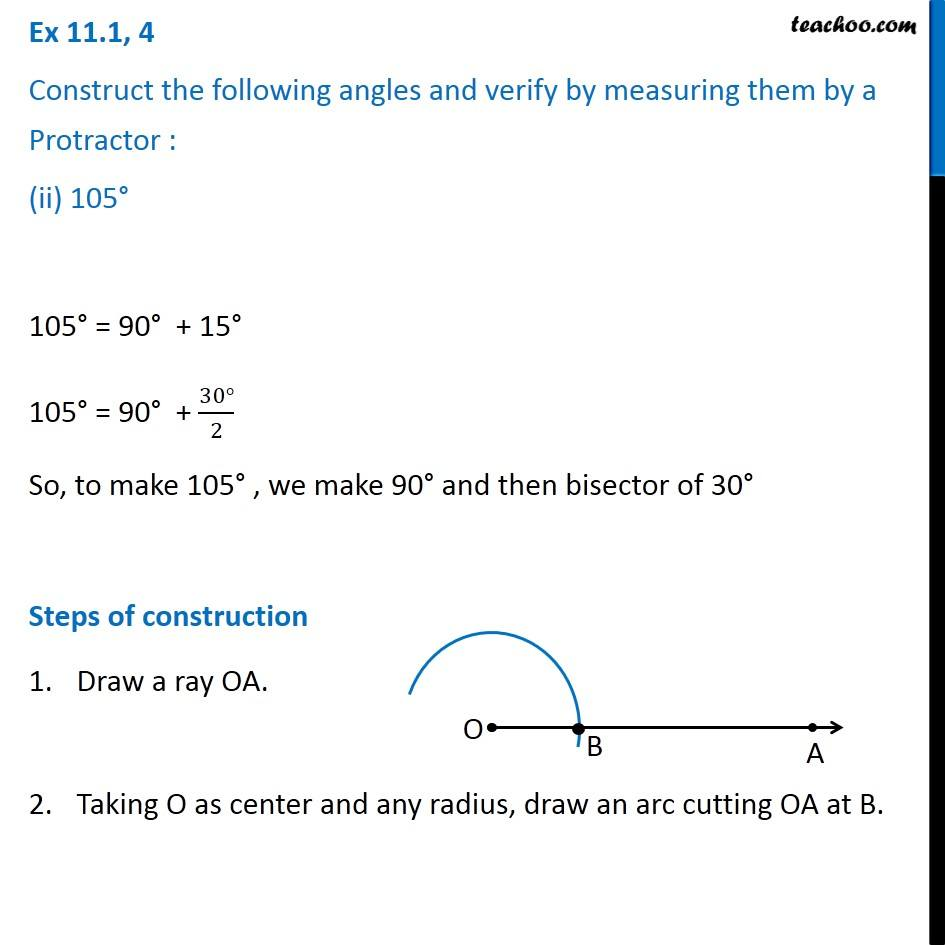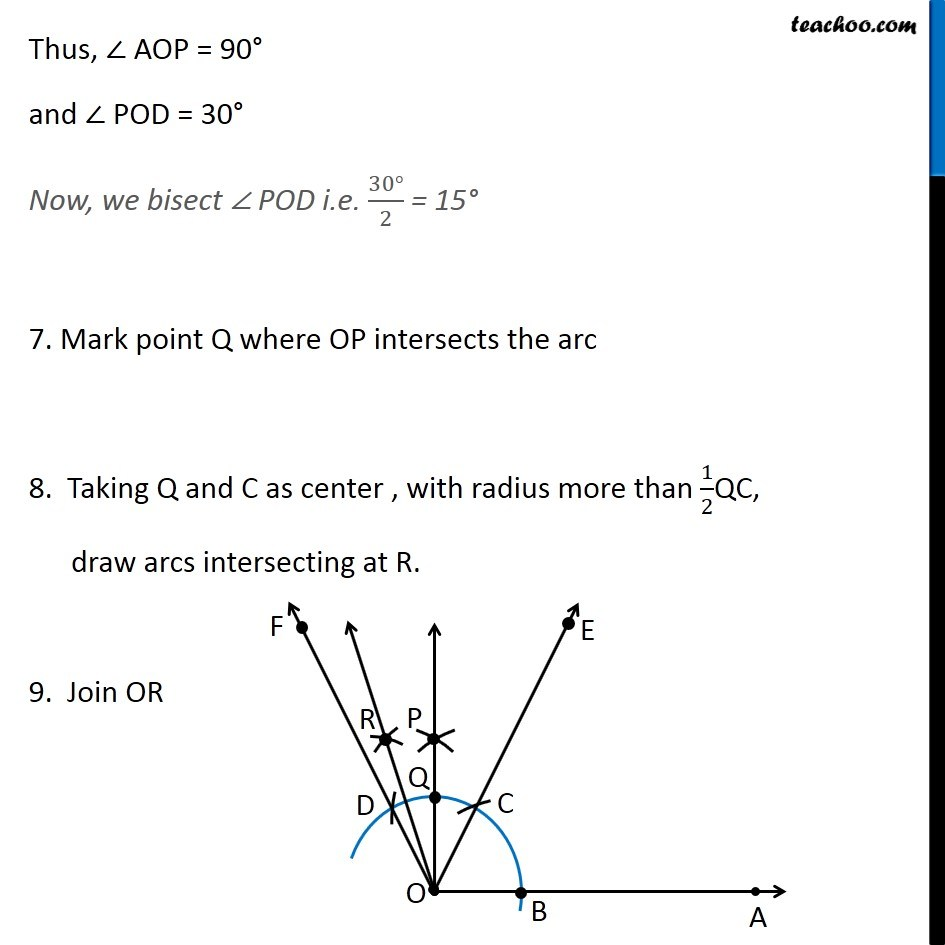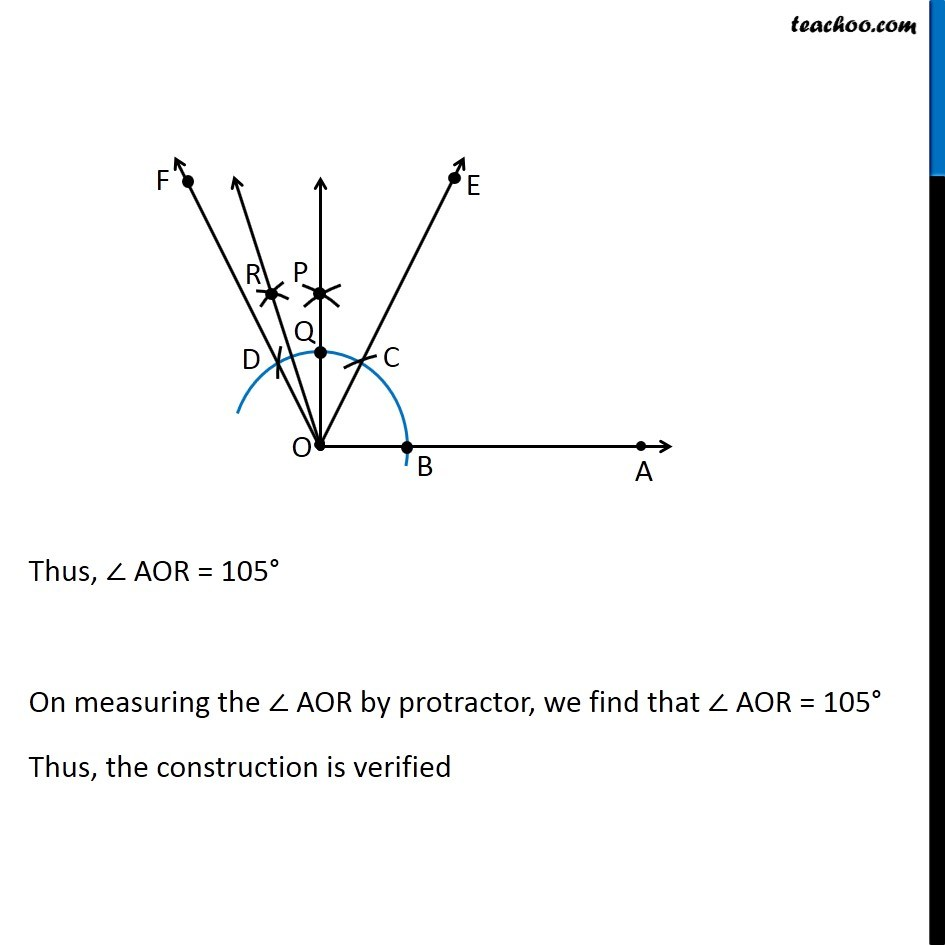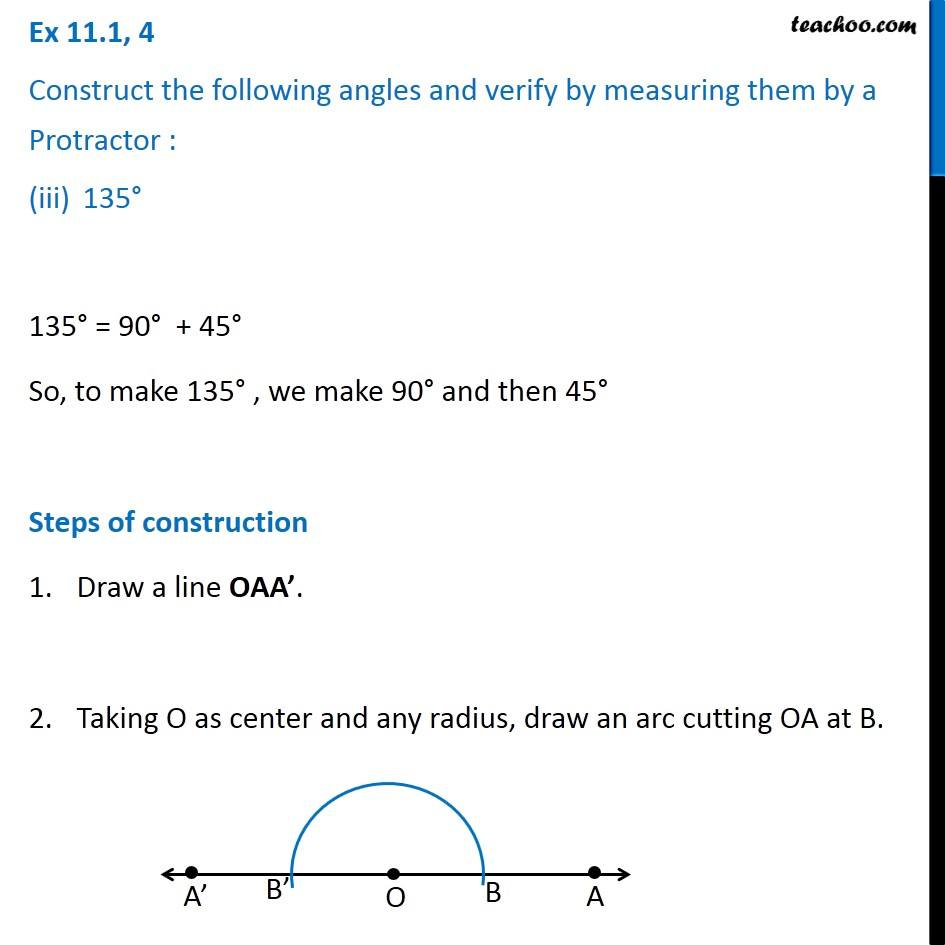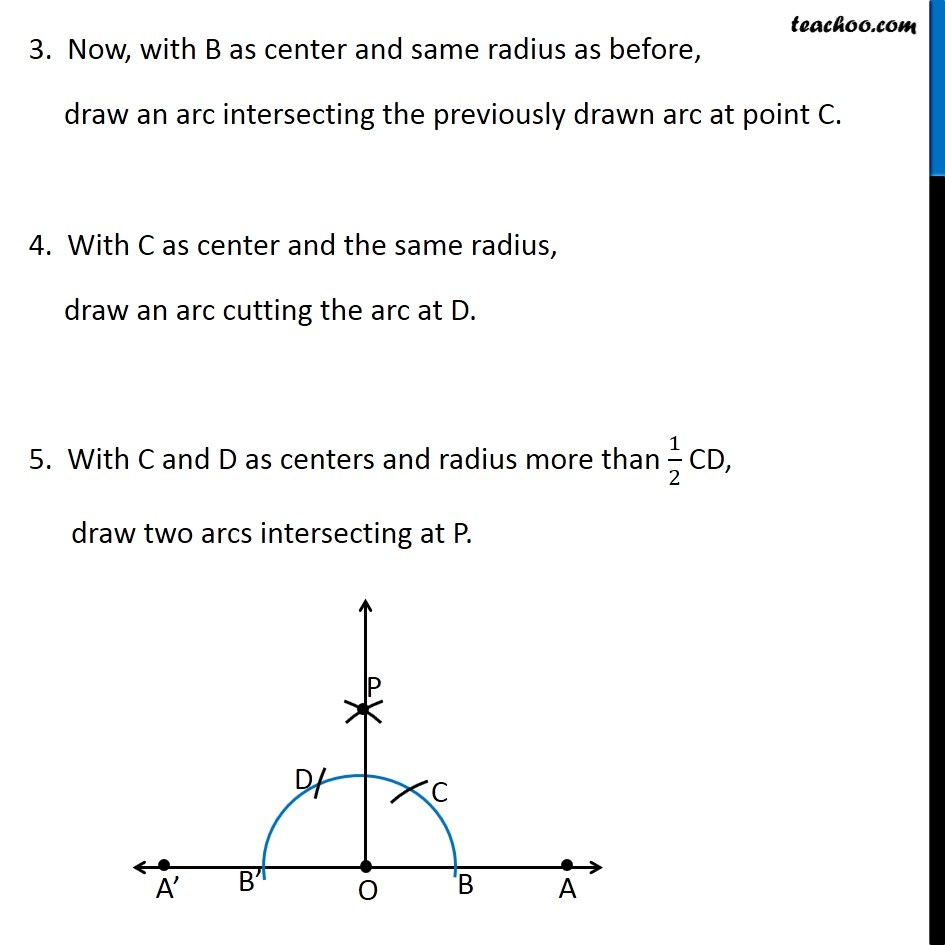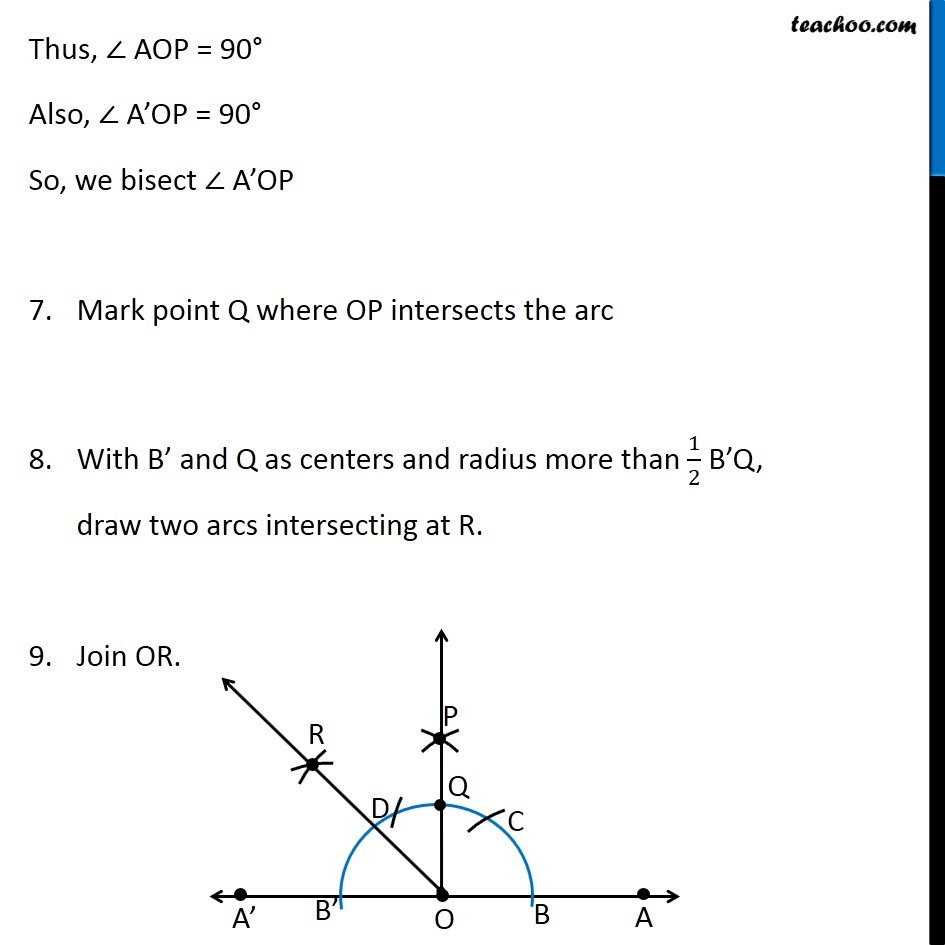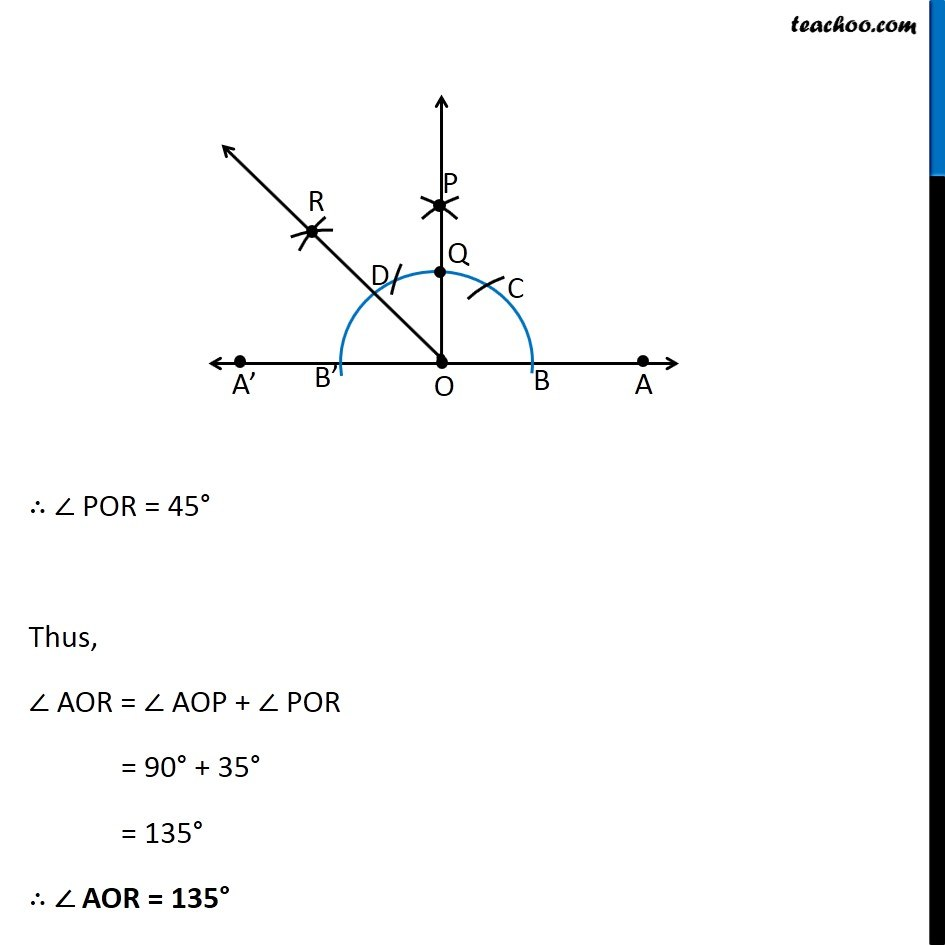1. Chapter 11 Class 9 Constructions
2. Serial order wise
3. Ex 11.1

Transcript

Ex 11.1, 4 Construct the following angles and verify by measuring them by a Protractor : 75° 75° = 60° + 15° 75° = 60° + (30°)/2 So, to we make 75° , we make 60° and then bisector of 30° Steps of construction Draw a ray OA. Taking O as center and any radius, draw an arc cutting OA at B. 3. Now, with B as center and same radius as before, draw an arc intersecting the previously drawn arc at point C. 4. Now, with C as center, and same radius, draw another arc intersecting the previously drawn arc at point D 5. Draw ray OE passing through C and ray OF passing through D Thus, ∠ AOE = 60° and ∠ EOF = 60° Now we bisect ∠ EOF twice 60° → 30° → 15° 6. Taking C and D as center , with radius more than 1/2CD, draw arcs intersecting at P. 7. Join OP Thus, ∠ EOP = 30° Now, we bisect ∠ EOP i.e. (30°)/2 = 15° 7. Mark point Q where OP intersects the arc 8. Taking Q and C as center , with radius more than 1/2QC, draw arcs intersecting at R. 9. Join OR Thus, ∠ AOR = 75° On measuring the ∠ AOR by protractor, we find that ∠ AOR = 75° Thus, the construction is verified Thus, ∠ AOR = 75° On measuring the ∠ AOR by protractor, we find that ∠ AOR = 75° Thus, the construction is verified Steps of construction Draw a ray OA. Taking O as center and any radius, draw an arc cutting OA at B. 3. Now, with B as center and same radius as before, draw an arc intersecting the previously drawn arc at point C. 4. Now, with C as center, and same radius, draw another arc intersecting the previously drawn arc at point D 5. Draw ray OE passing through C and ray OF passing through D Thus, ∠ AOE = 60° and ∠ EOF = 60° Now we bisect ∠ EOF twice 60° → 30° → 15° 6. Taking C and D as center , with radius more than 1/2CD, draw arcs intersecting at P. 7. Join OP Thus, ∠ AOP = 90° and ∠ POD = 30° Now, we bisect ∠ POD i.e. (30°)/2 = 15° 7. Mark point Q where OP intersects the arc 8. Taking Q and C as center , with radius more than 1/2QC, draw arcs intersecting at R. 9. Join OR Thus, ∠ AOP = 90° and ∠ POD = 30° Now, we bisect ∠ POD i.e. (30°)/2 = 15° 7. Mark point Q where OP intersects the arc 8. Taking Q and C as center , with radius more than 1/2QC, draw arcs intersecting at R. 9. Join OR Ex 11.1, 4 Construct the following angles and verify by measuring them by a Protractor : 135° 135° = 90° + 45° So, to make 135° , we make 90° and then 45° Steps of construction Draw a line OAA’. Taking O as center and any radius, draw an arc cutting OA at B. 3. Now, with B as center and same radius as before, draw an arc intersecting the previously drawn arc at point C. 4. With C as center and the same radius, draw an arc cutting the arc at D. 5. With C and D as centers and radius more than 1/2 CD, draw two arcs intersecting at P. Thus, ∠ AOP = 90° Also, ∠ A’OP = 90° So, we bisect ∠ A’OP Mark point Q where OP intersects the arc With B’ and Q as centers and radius more than 1/2 B’Q, draw two arcs intersecting at R. Join OR. ∴ ∠ POR = 45° Thus, ∠ AOR = ∠ AOP + ∠ POR = 90° + 35° = 135° ∴ ∠ AOR = 135°

Ex 11.1

About the AuthorDavneet Singh
Davneet Singh is a graduate from Indian Institute of Technology, Kanpur. He has been teaching from the past 9 years. He provides courses for Maths and Science at Teachoo.